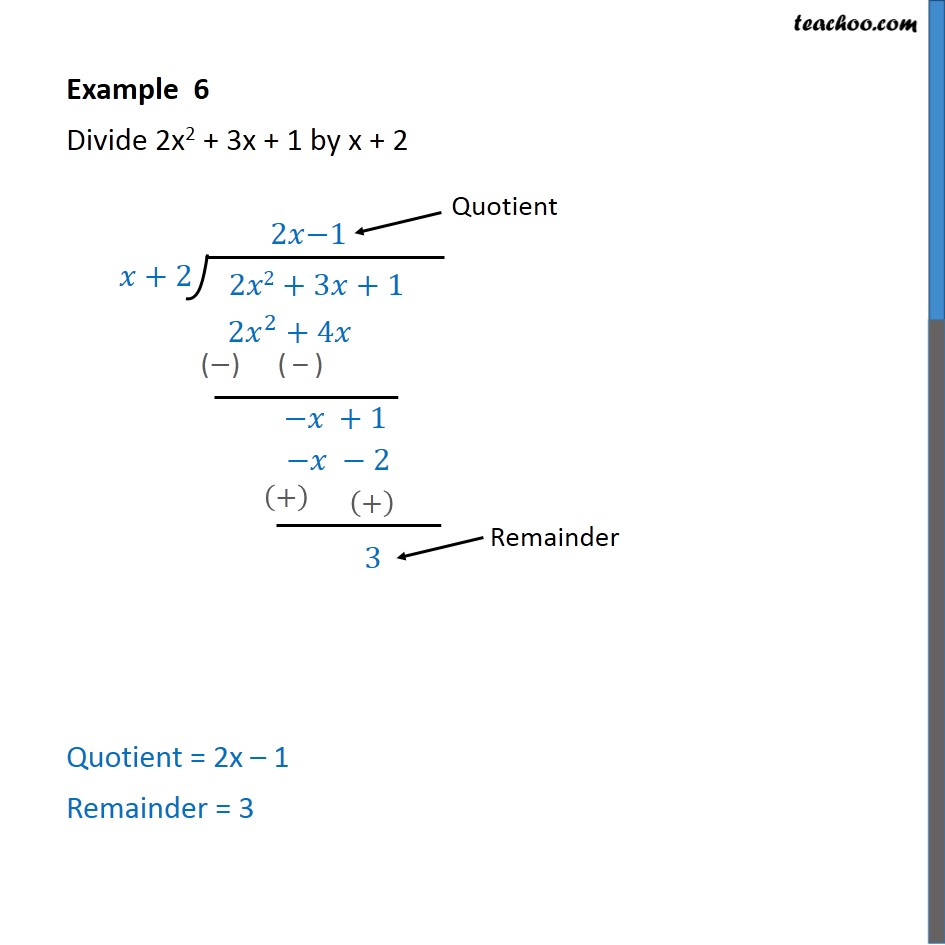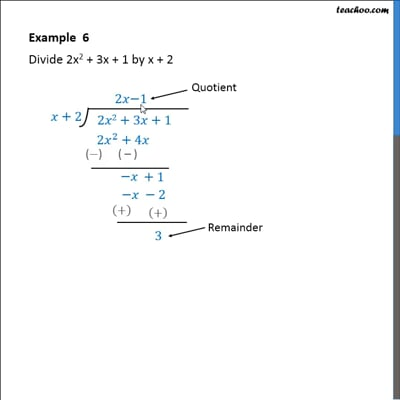Examples

Chapter 2 Class 10 Polynomials
Serial order wiseThis video is only available for Teachoo black users

Maths Crash Course - Live lectures + all videos + Real time Doubt solving!

### Transcript

Example 6 Divide 2x2 + 3x + 1 by x + 2 We follow these steps 1. Since it is 2x2, we multiply x by 2x 2. So, our remainder will be difference of 2x2 + 3x and 2x2 + 4x 3. Which comes out to be -x 4. So, we muliply by -1 5. The remainder will be the difference of -x + 1 and -x -2. 5. Which comes out to be +3 Thus, Quotient = 2x – 1 Remainder = 3